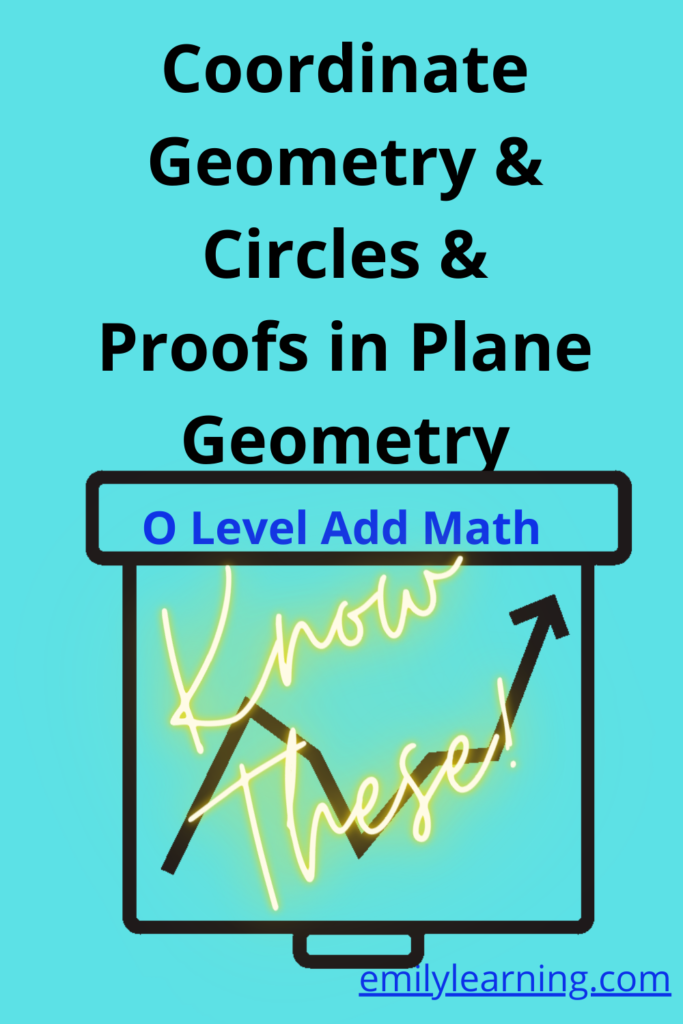# What you need to know for coordinate geometry, circles and proofs in plane geometry tested in O Level Additional MathematicsLet’s talk about what you need to know for Coordinate geometry, circles (in coordinate geometry) and proofs in plane geometry for O Level Additional Mathematics.

### Coordinate geometry

• finding the gradient of a straight line from 2 given points
• finding distance between 2 points
• finding the midpoint of 2 points
• finding the equation of a straight line
• relationship between gradients when the straight lines are parallel or perpendicular
• finding the equation of the perpendicular bisector
• finding area using shoe-lace method

### Circles in coordinate geometry

• equation of circles in the following form:
• (x-a)2+ (y-b)2 =r2
• x2 + y2+ 2gx + 2fy +c = 0
• problems involving circles and coordinate geometry

### Proofs in plane geometry

• proofing similar and congruent triangle (also tested in elementary mathematics)
• mid-point theorem
• alternate angles and corresponding angles
• alternate segment theorem and other geometric properties of circles (e.g. angle in the same segment, angle in a semi- circle, the angle at the centre of circle, etc.)

## Want to learn O Level Additional Math on-demand?

Check out our on-demand O Level Additional Math course here.

error: Content is protected !!